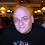## VizWiz

Launch, grow, and unlock your career in data

# Tableau Tip Tuesday: Using the Modulo Function to Conditionally Format NumbersThis week's tip came from a question reader Jonathan Hayter sent me.

I want to show one decimal point on the value of my mark labels -- except when the digit following the decimal point is zero. So, in the example below, I'd like to make the label for 2013 show as "57%" rather than "57.0%."

Great question! I told Jonathan that I disagree with formatting the numbers differently, but I also wanted to help him find a solution. The answer is two calculations and the modulo function.

In the final version you see below I've included a third calculation to highlight the bars that a zero following the decimal point. This is merely to make it easier for you to see. I didn't show this in the video. Download the workbook to see the solution.

Enjoy!

1.Thanks, Andy! Works like a charm. I was not familiar with the Modulo function. Seems like it could be helpful in a number of ways.

2.Just to finish it off, you can apply the same logic in to tool tip as well!

Profit Ratio:

3.Nice tip! I've sometimes run into problems with calcs using the modulo function (as in the underlying data source can't compute them), an alternative formula that doesn't require modulo would be IF ([Profit Ratio]*100)-INT([Profit Ratio]*100)= 0 THEN [Profit Ratio] END. The idea here is that if the (value - rounded down value) equals 0 then the value must be a whole number.

Jonathan

1.Ah good one there Jonathan!

4.